Home > CAAC > Chapter 7 > Lesson 7.3.4 > Problem7-115

7-115.
1. Explain what the slope of each line below represents. Then find the slope. Homework Help ✎

1.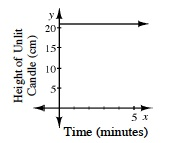2.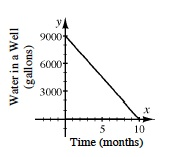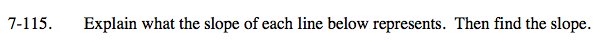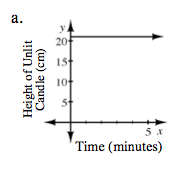The slope represents the change in height of a candle per minute.

The line is horizontal and shows no change in slope; therefore, the slope is zero and the candle is not burning.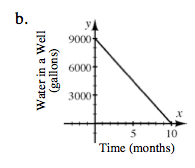The slope represents the gallons per month of water being removed from a well.

Using a slope triangle, find the distances of both legs of the triangle and solve in the slope formula.

$\text{Slope}=\frac{\Delta y}{\Delta x}$